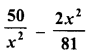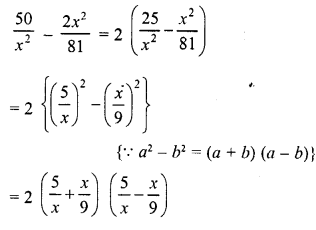# RD Sharma Class 8 Solutions Chapter 7 Factorizations Ex 7.5

In this chapter, we provide RD Sharma Class 8 Solutions Chapter 7 Factorization Ex 7.5 for English medium students, Which will very helpful for every student in their exams. Students can download the latest RD Sharma Class 8 Solutions Chapter 7 Factorization Ex 7.5 Maths pdf, free RD Sharma Class 8 Solutions Chapter 7 Factorization Ex 7.5 Maths book pdf download. Now you will get step by step solution to each question.

### RD Sharma Solutions for Class 8 Chapter 7 Factorization Ex 7.5Download PDF

Factorize each of the following expressions :
Question 1.
16x2-25y2
Solution:
16x2 – 25y2 = (4x)2 – (5y)2    {∵ a2 – b2 = (a + b) (a – b)}
= (4x + 5y) (4x – 5y)

Question 2.
27x2 – 12y2
Solution:
27x2 – 12y2 = 3 (9x2 – 4y2)  {∵ a2 -b2 = (a + b) (a – b)}
= 3 [(3x)2 – (2y)2]
= 3 (3x + 2y) (3x – 2y)

Question 3.
144a– 289b2
Solution:
144a2 – 289b2 = (12a)2 – (17b)2    { ∵ a2 – b2 = (a + b) (a – b}
= (12a+ 17b) (12a- 17b)

Question 4.
12m2 – 27

Solution:
12m2 – 27 = 3 (4m2 – 9)
= 3 {(2m)2-(3)2}   {∵ a2 – b2 = (a + b) (a – b)}
= 3 (2m + 3) (2m – 3)

Question 5.
125x2 – 45y2
Solution:
125x2 – 45y2 = 5 (25x2 – 9y2)
= 5 {(5x-)2 – (3y)2}    {∵ a2 – b2 = (a + b) (a – b}
= 5 (5x + 3y) (5x – 3y)

Question 6.
144a2 – 169b
2
Solution:
144a2 – 169b2 = (12a)2 – (13b)2    {∵ a2 -b2 = (a + b) (a – b)}
= (12a + 13b) (12a-13b)

Question 7.
(2a – b)2 – 16c2
Solution:
(2a – b)2 – 16c2 = (2a – b)2 – (4c)2   {∵ a2 – b2 = (a + b) (a – b)}
= (2a – b + 4c) (2a – b – 4c)

Question 8.
(x + 2y)2 – 4 (2x -y)2
Solution:
(x + 2y)2 – 4 (2x – y)2
= (x + 2y)2 – {2 (2x –y)}2
= (x + 2y)2 – (4x – 2y)2        {∵ a2– b2 = (a + b) (a – b)}
= (a + 2y + 4x – 2y) (x + 2y – 4x + 2y)
= 5x (-3x + 4y)

Question 9.
3a5 – 48a3
Solution:
3a5 – 48a3 = 3a3 (a2– 16)
= 3a3 {(a)2 – (4)2}        {∵ a2 – b2 = (a + b) (a – b)}
= 3a3 (a + 4) (a – 4)

Question 10.
a4 – 16b4
Solution:
a4 – 16b4 = (a2)2 – (4b2)2
= (a2 + 4b2) (a2 – 4b2)
= (a2 + 4b2) {(a)2 – (2b)2 }   { ∵ a2 – b2 = (a + b) (a – b)}
= (a2 + 4b2) (a + 2b) (a – 2b

Question 11.
x8 – 1
Solution:
x8 – 1 = (x4)2 – (1)2
= (x4 + 1) (x4 – 1)
= (x4+ 1) I (x2)2 – (1)2}             {∵ a2 – b2 = (a + b) (a – b)}
= (x4 + 1) (x2 + 1) (x2 – 1)
= (x4 + 1) (x2 + 1) {(x)2 – (1)2}
= (x4+ 1)(x2 + 1)(x+ 1)(x- 1)
= (x-1)(x+ 1) (x2 + 1) (x4 + 1)

Question 12.
64 – (a + 1)2
Solution:
64 – (a + 1)2 = (8)2 – (a + 1)2    {∵ a2 – b2 = (a + b) (a – b)}
= (8 + a + 1) (8 – a – 1)
= (9 + a) (7 – a)

Question 13.
36l2 – (m + n)2
Solution:
36l2 – (m + n)2 = (6l)2 – (m + n)2        {∵  a2 – b2 = (a + b) (a – b)}
= (6l + m + n) (6l – m – n)

Question 14.
25x4y4 – 1
Solution:
25x4y4 – 1 = (5x4y4)2 – (1)2         { ∵  a2 – b2 = (a + b) (a – b)}
= (5x4y4  + 1) (5x2y2  – 1)

Question 15.Solution:Question 16.
x3 – 144x
Solution:
x3 – 144x = x (x2 – 144)
= x {(x)2 – (12)2}       {∵ a2 – b2 = (a + b) (a – b)}
=  x (x + 12) (x – 12)

Question 17.
(x – 4y)2
– 625
Solution:
(x – 4y)2 – 625
= (x – 4y)2 – (25)2     {∵ a2 – b2 = (a + b) (a – b)}
= (x – 4y + 25) (x -4y – 25)

Question 18.
9 (a – b)2 – 100 (x -y)2
Solution:
9(a-b)2– 100(x-y)2
= {3(a-b)}2-{10(x-y)}2      {∵ a2 – b2 = (a + b) (a – b)}
= (3a – 3b)2 – (10x – 10y)2
= (3a – 3b + 10x – 10y) (3a – 3b – 10x + 10y)

Question 19.
(3 + 2a)2 – 25a2
Solution:
(3 + 2a)2 – 25a2
= (3 + 2a)2 – (5a)2      (∵ a2 – b2 = (a + b) (a – b)}
= (3 + 2a + 5a) (3 + 2a – 5a)
= (3 + 7a) (3 – 3a)
= (3 + 7a) 3 (1 – a)
= 3(1-a) (3 +7a)

Question 20.
(x + y)2 – (a – b)2
Solution:Question 21.Solution:Question 22.
75a3b2 – 108ab4
Solution:
75a3b2 – 108ab4
= 3ab2 (25a2 – 36b2)
= 3ab2 {(5a)2 – (6b)2}         {∵ a2 – b2 = (a + b) (a – b)}
= 3ab2 (5a + 6b) (5a – 6b)

Question 23.
x5– 16x3
Solution:
x5 – 16x3 = x3 (x2 – 16)
= x3 {(x)2 – (4)2} {∵ a2 – b2 = (a + b) (a – b)}
= x3 (x + 4) (x – 4)

Question 24.Solution:Question 25.
256x5 – 81x
Solution:
256x5– 81x = x(256x4– 81)
= x {(16x2)2 – (9)2}      {∵ a2 – b2 = {a + b) (a – b)}
= x (16x2 + 9) (16x2 – 9)
= x (16x2 + 9) {(4x)2 – (3)2}
= x (16x2 + 9) (4x + 3) (4x-3)

Question 26.
a4 – (2b + c)
4
Solution:
a4 – (2b + c)4
= (a2)2 – [(2b + c)2]2    {∵ a2 – b2 = (a + b) (a – b)}
= {a2 + (2b + c)2} {a2 – (2b + c)2}
= {a2 + (2b + c)2} {(a)2 – (2b + c)2}
= {a2 + (2b + c)2} (a + 2b + c) (a -2b- c)

Question 27.
(3x + 4y)4 – x4
Solution:
(3x + 4y)4 – x4 – [(3x + 4y)2]2 – (x2)2
= [(3x + 4y)2 + x2] [(3x + 4y)2 – x2]       {∵  a2 – b2 = (a + b) (a – b)
= [(3x + 4y)2 + x2] [(3x + 4y + x) (3x + 4y – x)]
=   [(3x + 4y)2 + x2] (4x + 4y) (2x + 4y)
= [(3x + 4y)2 + x2] 4 (x + y) 2 (x + 2y)
= 8 (x + y) (x + 2y) [(3x + 4y)2 + x2]

Question 28.
p2q2 – p4q4
Solution:
p2q2– p4q=p2q2 (1 -p2q2)
=p2q2 [(1)2 – (pq)2]   {∵ a2 – b2 = (a + b) (a – b)
= p2q2 (1 +pq) (1 -pq)

Question 29.
3x3y – 243xy3
Solution:
3x3y – 243xy3
= 3xy (x2 – 81y2)
= 3xy [(x)2 – (9y)2]
= 3xy (x + 9y) (x – 9y)

Question 30.
a4b4 – 16c4
Solution:
a4b4 – 16c4 = (a2b2)2 – (4c2)2
= (a2b2 + 4c2) (a2b2 – 4c2)
= (a2b2 + 4c2) [(ab)2 – (2c)2]      {∵ a2 – b2 = (a + b) (a – b)
= (a2b2 + 4c2) (ab + 2c) (ab – 2c)

Question 31.
x4-625
Solution:
x4 – 625 = (x2)2 – (25)2   {∵ a2 – b2 – (a + b) (a – b)
= (x2 + 25) (x2 – 25)
= (x2 + 25) [(x)2 – (5)2]
= (x2 + 25) (x + 5) (x – 5)

Question 32.
x4-1
Solution:
x4 – 1 = (x2)2 – (1)2 = (x2 + 1) (x2 – 1)
= (x2 + 1) [(x)2 – (1)2]
= (x2 + 1) (x + 1) (x – 1)

Question 33.
49 (a – b)2 -25 (a + b)2
Solution:
49 (a – by -25 (a + b)2
= [7 (a – b)]2 – [5 (a + b)]2
= (7a – 7b)2 – (5a + 5b)2  {∵ a2 – b2 = (a + b) (a – b)
= (7a -7b + 5a + 5b) (7a – 7b -5a- 5b)
=(12a – 2b)(2a – 12b)
= 2 (6a – b) 2 (a – 6b)
= 4 (6 a- b) (a – 6b)

Question 34.
x – y – x2 + y
Solution:
x-y-x2 + y= (x-y)-(x2-y2) {∵ a2 – b2 = (a + b) (a – b)
= {x-y)-(x + y)(x-y)
= (x-y)(1 – x – y)

Question 35.
16 (2x – 1)2 – 25y2
Solution:
16 (2x – 1)2 – 25y2
= [4 (2x – 1)]2 – (5y)2
= (8x – 4)2 – (5y)2
= (8x – 4 + 5y) (8x -4-5y)
= (8x + 5y – 4) (8x – 5y – 4)

Question 36.
4 (xy + 1)2 – 9 (x – 1)2
Solution:
4 (xy + 1)2 – 9 (x – 1)2
=
[2 (xy + 1)]2 – [3 (x – 1)]2
= (2xy + 2)2 – (3x – 3){∵ a2 – b2 = (a + b) (a – b)
= (2xy + 2 + 3x – 3) (2xy + 2 – 3x + 3)
= (2xy + 3x – 1) (2xy – 3x + 5)

Question 37.
(2x + 1)2 – 9x4
Solution:
(2x + 1)2 – 9x4 = (2x + 1)2 – (3x2)2    {∵ a2 – b2 = (a + b) (a – b)
= (2x + 1 + 3x2) (2x + 1 – 3x2)
= (3x2 + 2x + 1) (-3x + 2x + 1)

Question 38.
x4 – (2y- 3z)2
Solution:
x4 – (2y – 3z)2 = (x2)2 – (2y – 3z)2= (x2 + 2y- 3z) (x2 – 2y + 3z)

Question 39.
a2-b2 +a-b
Solution:
a2 – b2 + a – b
= (a + b) {a – b) + 1 (a – b)
= (a – b) (a + b + 1)

Question 40.
16a4 – b4
Solution:
16a4 – b4
= (4a2)2 – (b2)2            { ∵  a2 – b2 = (a + b) (a – b)
= (4a2 + b2) (4a2 – b2)
= (4a2 + b2) {(2a)2 – (b)2}
= (4a2 + b2) (2a + b) (2a – b)

Question 41.
a4 – 16 (b – c)4
Solution:
a4 – 16 (b- c)4 = (a2)2 – [4 (b – c)2]{ ∵  a2 – b2 = (a + b) (a – b)
= [a2 + 4 (b – c)2] [a2 – 4 (b – c)2]
= [a2 + 4 (b – c)2] [(a)2 – [2 (b – c)]2]
= [a2 + 4 (b – c)2] [(a)2 – (2b – 2c)2]
= [a2 + 4 (b – c)2] (a + 2b – 2c) (a – 2b + 2c)

Question 42.
2a5 – 32a
Solution:
2a5 – 32a = 2a (a4 – 16)
= 2a [(a2)2 – (4)2]  {∵  a2 – b2 = (a + b) (a – b)
= 2a (a2 + 4) (a2 – 4)]
= 2a (a2 + 4) [(a)2 – (2)2]
= 2a (a2 + 4) (a + 2) (a – 2)

Question 43.
a4b4 – 81c4
Solution:
a4b4 – 81c4 = (a2b2)2 – (9c2)2
= (a2b2 + 9c2) (a2b2 – 9c2) {∵ a2 – b2 = (a + b) (a – b)
= (a2b2 + 9c2) {(ab)2 – (3c)2}
= (a2b2 + 9c2) (ab + 3c) (ab – 3c)

Question 44.
xy9-yx9
Solution:
xy9 – yx9 = xy (y8 – x8)
= xy [(y4)2 – (x4)2] {∵  a2 – b2 = (a + b) (a – b)}
= xy(y4 + x4)(y4-x4)
= xy (y4 + x4) {(y2)2 – (x2)2}
= xy (y4 + x4) (y2 + x2) (y2 – x2)
= xy (y4 + x4) (y2 + x2) (y + x) (y – x)

Question 45.
x3 -x
Solution:
x3-x = x(x2– 1)
= x [(x)2 – (1)2] = x (x + 1) (x – 1)

Question 46.
18a2x2 – 32

Solution:
18a2x2 – 32
= 2 [9a2x2 – 16]
= 2 [(3ax)2 – (4)2]   {∵ a2 – b2 = (a + b) (a – b)
= 2 (3ax + 4) (3ax – 4)

All Chapter RD Sharma Solutions For Class 8 Maths

—————————————————————————–

All Subject NCERT Exemplar Problems Solutions For Class 8

All Subject NCERT Solutions For Class 8

*************************************************

I think you got complete solutions for this chapter. If You have any queries regarding this chapter, please comment on the below section our subject teacher will answer you. We tried our best to give complete solutions so you got good marks in your exam.

If these solutions have helped you, you can also share rdsharmasolutions.in to your friends.